# Wiener sausage

(diff) ← Older revision | Latest revision (diff) | Newer revision → (diff)

Let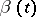,, be the standard Brownian motion in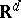(i.e. the Markov process with generator) starting at. Let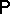,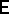denote its probability law and expectation on path space. The Wiener sausage with radius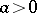is the process defined bywhere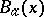is the open ball with radiusaround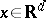.

The Wiener sausage is an important mathematical object, because it is one of the simplest examples of a non-Markovian functional of Brownian motion. It plays a key role in the study of various stochastic phenomena, such as heat conduction and trapping in random media, as well as in the analysis of spectral properties of random Schrödinger operators (cf. also Schrödinger equation).

A lot is known about the behaviour of the volume ofas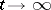. For instance,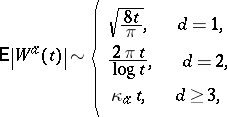with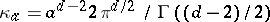the Newtonian capacity of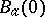associated with the Green's function of(cf. also Green function; Capacity), and([a8], [a6]). Moreover,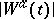satisfies the strong law of large numbers and the central limit theorem for; the limit law is Gaussian for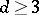and non-Gaussian for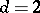([a7]). Note that for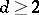the Wiener sausage is a sparse object: since the Brownian motion typically travels a distance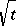in each direction, the last two displays show that most of the space in the convex hull of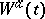is not covered.

The large deviation behaviour ofin the downward direction has been studied in [a5], [a4] and [a9]. Forthe outcome, proved in successive stages of refinement, reads as follows: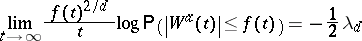for any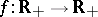satisfying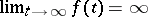and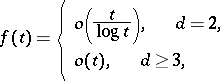where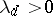is the smallest Dirichlet eigenvalue of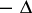on the ball with unit volume. The optimal strategy for the Brownian motion to realize the large deviation is to stay inside a ball with volumeuntil time, i.e., the Wiener sausage covers this ball entirely and nothing outside. This comes from the Faber–Krahn isoperimetric inequality (cf. also Rayleigh–Faber–Krahn inequality), and the cost of staying inside the ball is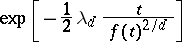to leading order. Note that, apparently, a large deviation below the scale of the mean "squeezes all the empty space out of the Wiener sausage" .

The above analysis of the large deviation behaviour has recently been extended to cover the moderate deviation behaviour. It is proved in [a2] that for,(a1)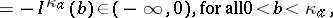and a variational representation is derived for the rate function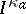. The optimal strategy for the Brownian motion to realize the moderate deviation is such that the Wiener sausage "looks like a Swiss cheese" :has random holes whose sizes are of orderand whose density varies on scale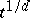. This is markedly different from the optimal strategy behind the large deviation. Note that, apparently, a moderate deviation on the scale of the mean "does not squeeze all the empty space out of the Wiener sausage" . (a1) has also been extended to.

It turns out that the rate function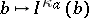exhibits rich behaviour as a function of the dimension. In particular, for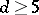it is non-analytic at a certain critical value inside, which is associated with a collapse transition in the optimal strategy.

Finally, the moderate and large deviations ofin the upward direction are a complicated issue. Here the optimal strategy is entirely different from the previous ones, because the Wiener sausage tries to expand rather than to contract. Partial results have been obtained in [a3] [a1], and [a11].

More background can be found in [a10].

How to Cite This Entry:
Wiener sausage. F. den Hollander (originator), Encyclopedia of Mathematics. URL: http://encyclopediaofmath.org/index.php?title=Wiener_sausage&oldid=11710
This text originally appeared in Encyclopedia of Mathematics - ISBN 1402006098